# Some Additional Distributions

Provides the density, distribution, quantile and generation functions of some obscure probability distributions, including the doubly non- central t, F, Beta, and Eta distributions; the lambda-prime and K-prime; the upsilon distribution; the (weighted) sum of non-central chi-squares to a power; the (weighted) sum of log non-central chi-squares; the product of non-central chi-squares to powers; the product of doubly non-central F variables; the product of independent normals.

Some Additional Distributions apparently not available in R.

-- Steven E. Pav, [email protected]

## Installation

This package can be installed from CRAN, via drat, or from github:

## Testing distributions

First some functions to test the 'dpqr' functions:

## Weighted sum of (non-central) chi-squares to power

This distribution is the weighted sum of independent (non-central) chi-square variates taken to some powers. The special case where the powers are all one half is related to the upsilon distribution. The special case where the powers are all one could be used to compute the distribution of the (doubly non-central) F distribution.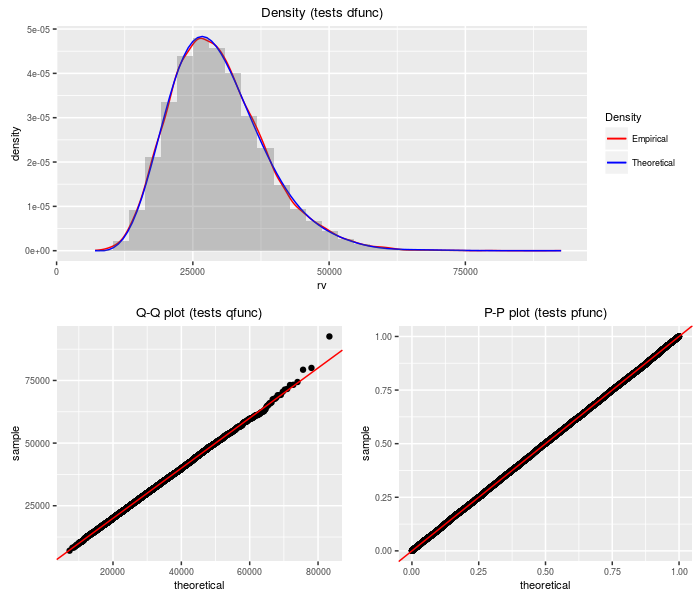## K-prime distribution

The K-prime distribution is the weighted sum of a standard normal and an independent central chi, all divided by another independent central chi. Depending on the degrees of freedom and the weights, the K-prime can appears as a Lambda-prime, a normal, or a central t.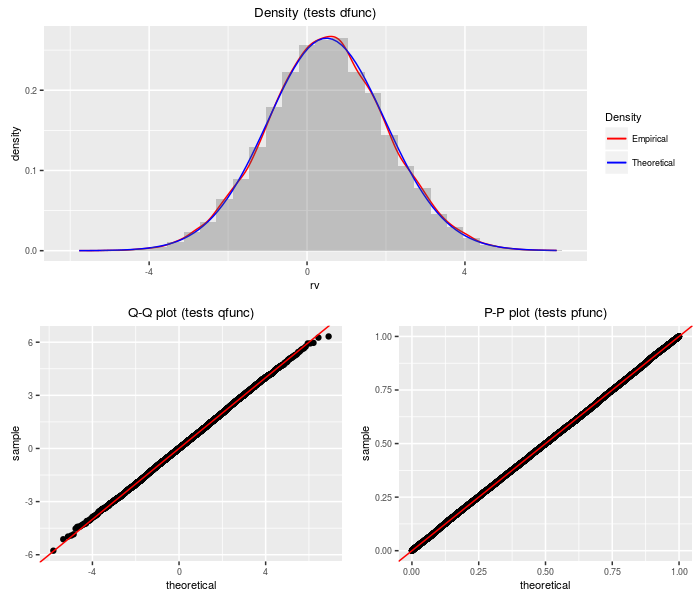## Lambda prime distribution

A Lambda prime random variable is the sum of a standard normal and an independent, scaled central chi random variable.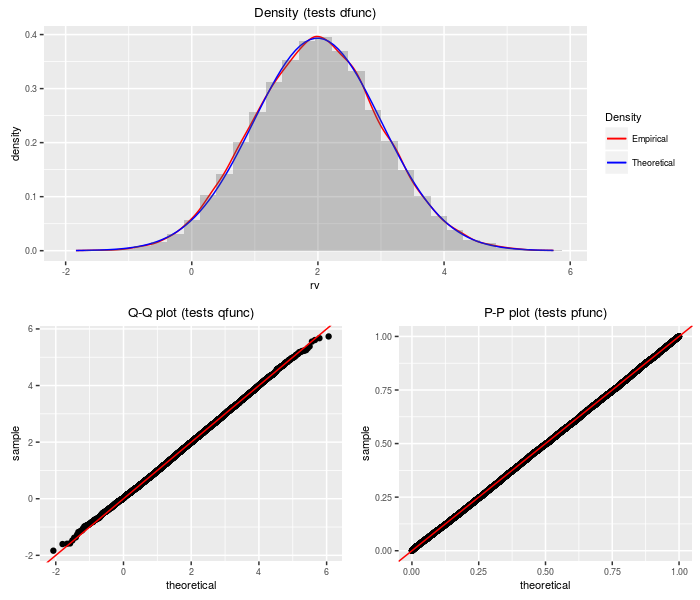## Upsilon distribution

An upsilon random variable is the sum of a standard normal and the weighted sum of several indpendent central chis.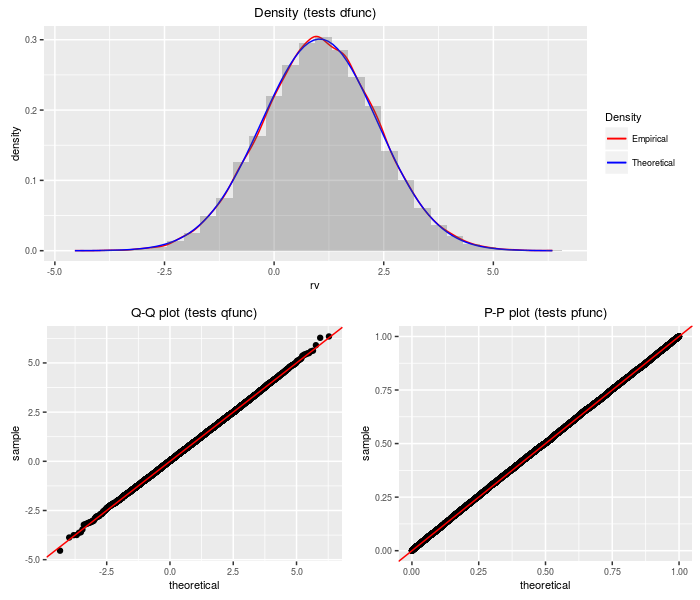## Doubly non-central t distribution

The doubly non-central t distribution generalizes the t distribution to the case where the denominator chi-square is non-central.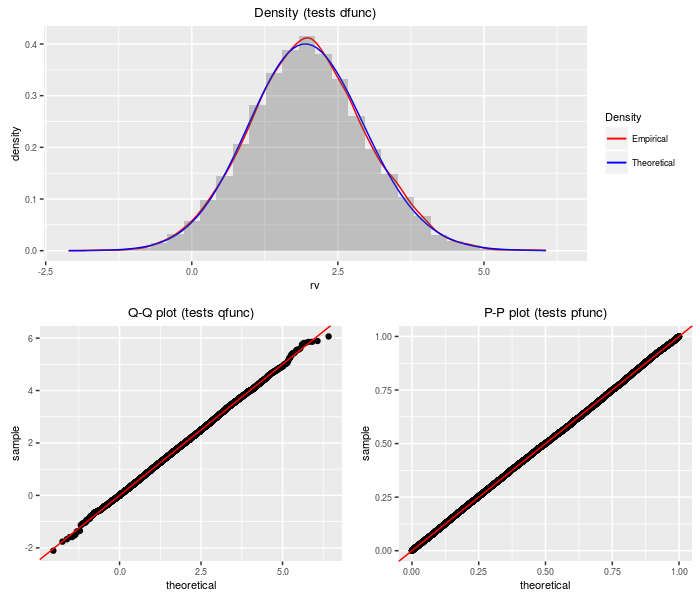## Doubly non-central F distribution

The doubly non-central F distribution generalizes the F distribution to the case where the denominator chi-square is non-central.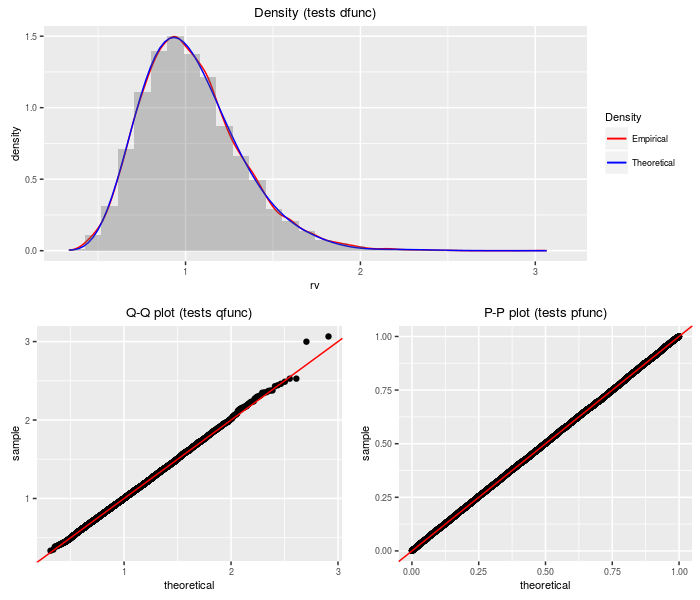## Doubly non-central Beta distribution

The doubly non-central Beta distribution can be viewed as a transformation of the doubly non-central F distribution.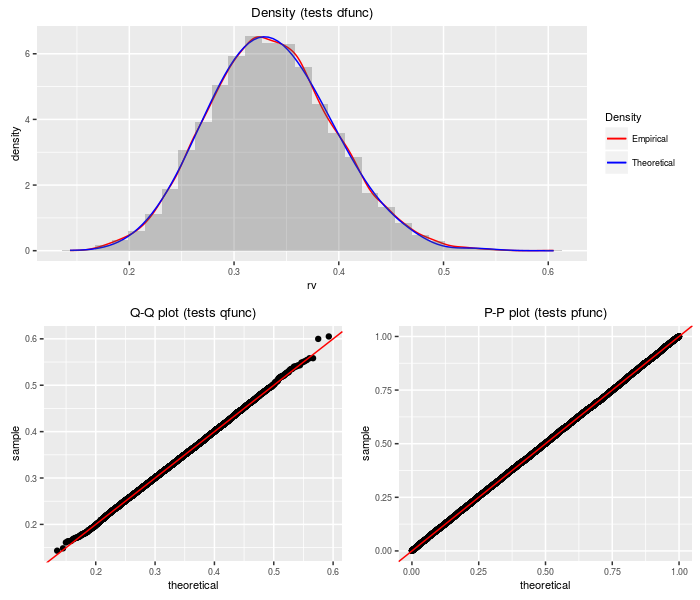## Doubly non-central Eta distribution

The doubly non-central Eta distribution can be viewed as the square root of the doubly non-central Beta distribution. It is a transform of the doubly non-central t distribution.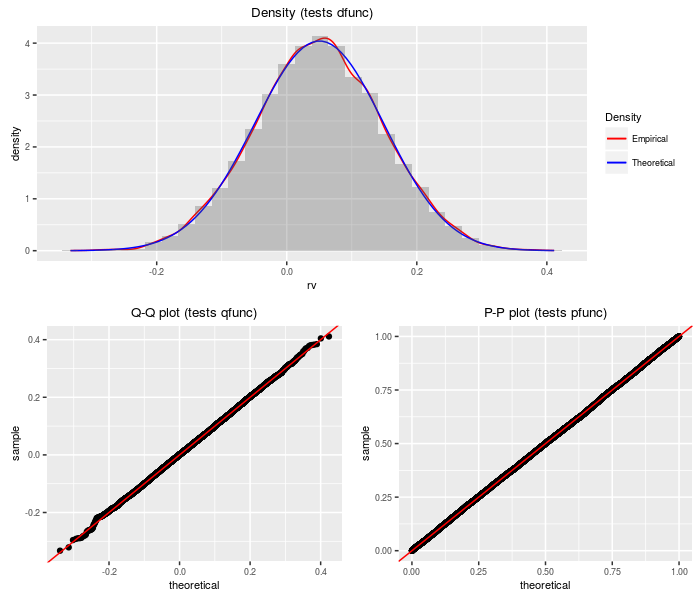## Weighted sum of logs of (non-central) chi-squares

This distribution is the weighted sum of logs of independent (non-central) chi-square variates.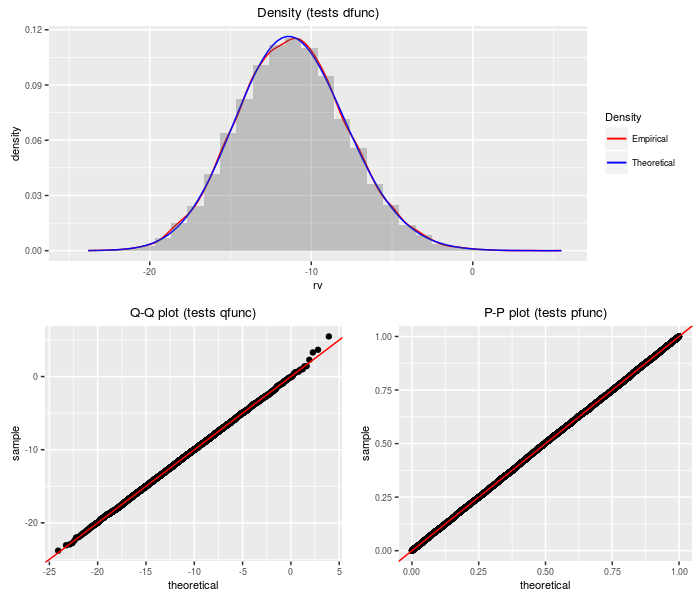## Product of doubly non-central F variates

This distribution is the product of independent doubly non-central F variates. The PDQ functions are computed by transformation on the sum of log chi-squares distribution.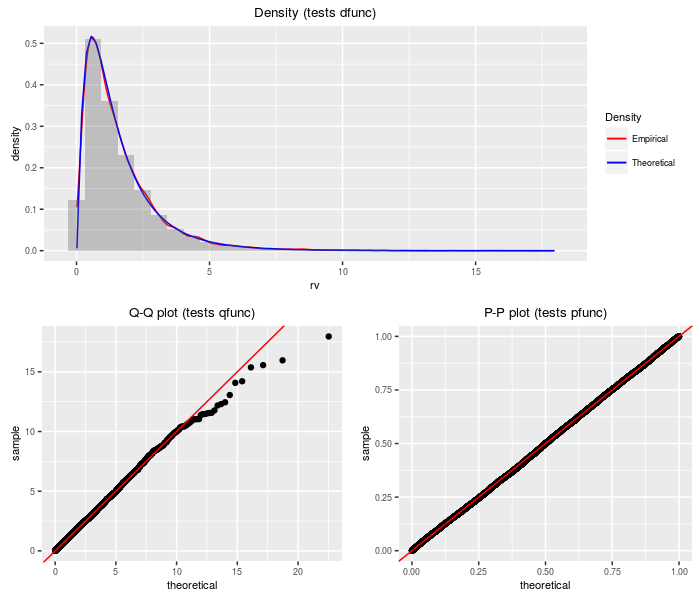## Product of (non-central) chi-squares to power

This distribution is the product of independent (non-central) chi-square variates taken to some powers. The PDQ functions are computed by transformation on the sum of log chi-squares distribution.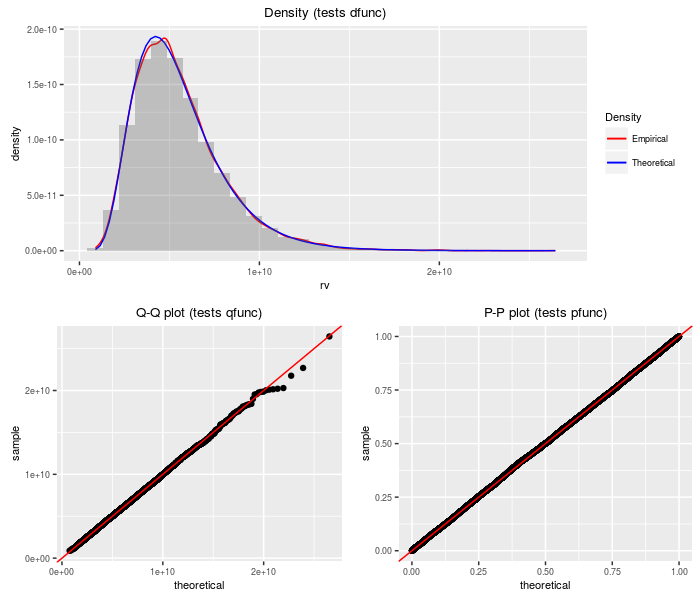## Product of independent normals

This distribution is the product of independent normal variates. Warning: when the coefficient of variation is large for some of the factors, this approximation can be terrible.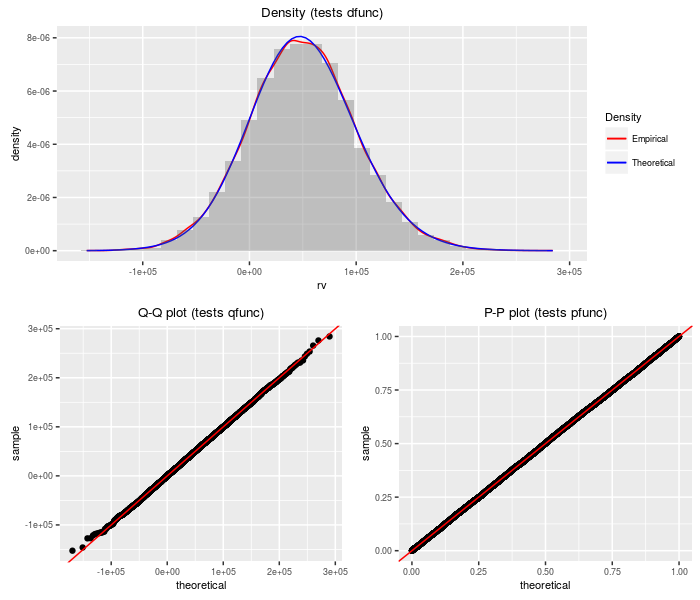# Reference manual

0.2.3 by Steven E. Pav, 2 years ago

Report a bug at https://github.com/shabbychef/sadists/issues

Browse source code at https://github.com/cran/sadists

Authors: Steven E. Pav [aut, cre]

Documentation:   PDF Manual

Task views: Probability Distributions